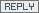## Saturday, April 5, 2008

### Is the car loan interest of 3% cheaper than house loan interest of 5%?

Many people are not aware that the interest calculation for car loan and house loan is different, so they cannot be compared directly figure by figure. If we compare the figure directly, is the car loan interest of 3% cheaper than house loan interest of 5%? You'll say 3% is definitely lower than 5%, right? However, the fact might be the other way round. 3% could be higher than 5%.

Let's do some calculation:

The total interest payable for a car loan of RM100k with interest 5% over a period of 9 years will be RM45,000. The monthly installment is RM1,342.60.

The total interest payable for a house loan of RM100k with interest 5% daily compounded over a period of 9 years will be RM24,439.20. The monthly installment is RM1,152.21.

The difference between the 2 calculations above is significant. Why? This is because car loan is calculated based on simple interest formula, and house loan is calculated based on amortized compound interest formula.

The formula for simple interest is straightforward, which is:

Interest = Principal x Rate x Years

What would be the car loan interest equilvalent to a house loan interest rate of 5%?

24,439.20 = 100,000 x Rate x 9
Rate = 2.72%

As a result, the above house loan interest rate of 5% is equilvalent to a car loan interest rate of 2.72%.

Is the car loan interest of 3% cheaper than house loan interest of 5% for the scenario above? The answer is obviously NO! However, there could be situation of the anwer to be yes too, especially when comparing a house loan of 30 years with a car loan of 9 years.

Here are some simple references for you:

For a 5 years 100k loan (the period where most people will take for car loan):
House loan interest of 4% = car loan interest of 2.10%
House loan interest of 5% = car loan interest of 2.65%
House loan interest of 6% = car loan interest of 3.21%
House loan interest of 7% = car loan interest of 3.77%
House loan interest of 8% = car loan interest of 4.35%
House loan interest of 9% = car loan interest of 4.93%

For a 9 years 100k loan (the maximum period for car loan):
House loan interest of 4% = car loan interest of 2.14%
House loan interest of 5% = car loan interest of 2.72%
House loan interest of 6% = car loan interest of 3.30%
House loan interest of 7% = car loan interest of 3.91%
House loan interest of 8% = car loan interest of 4.53%
House loan interest of 9% = car loan interest of 5.16%

For a 30 years 100k loan (the period where most people will take for house loan):
House loan interest of 4% = car loan interest of 2.40%
House loan interest of 5% = car loan interest of 3.12%
House loan interest of 6% = car loan interest of 3.87%
House loan interest of 7% = car loan interest of 4.67%
House loan interest of 8% = car loan interest of 5.49%
House loan interest of 9% = car loan interest of 6.35%

Anonymous said...What is the formula for calculating the interest for house loan?

Voyager8 said...Lee,

The formula is pretty complicated.

qhewei said...hi,
thanks for the detailed explanation, ur post has really cleared up my long-time confusion abt the much lower car loan rate !

btw, isn't the house loan monthly payment rm1151.72 ?
er..this is what i got after keying the info into my financial calculator....

Peter Lim said...A simple rule of thumb is Car loan rate is about 1.8 times the Housing Loan rate.

Thus, a 3% * 1.8 would equal 5.4% , which is higher than the 5% interest charged by the housing loan.

Do note that the 1.8 times is just an approximation, and not accurate figure.

My website is at http://peterlim80.blogspot.com